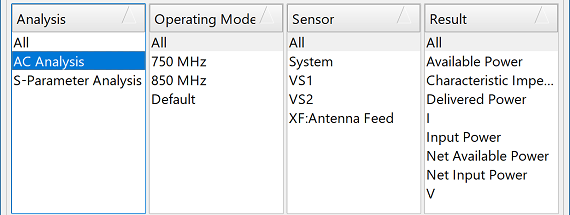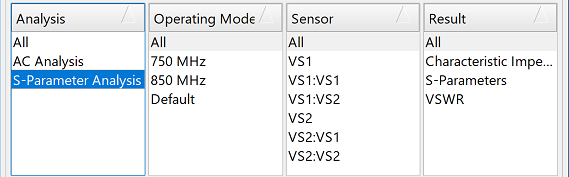Each execution of the frequency-domain circuit solver generates results for the current schematic, and the schematic's result browser allows those results to be filtered, selected, and viewed. The circuit solver performs both AC and S-parameter analyses and provides the associated results. Users can view a result by double-clicking in the lower portion of the schematic's results browser, or right-click to access additional options.

## AC AnalysisThe AC Analysis provides results for a schematic with all voltage sources active. Depending on the schematic's contents, five sensor types—FDTD port, voltage source, system, voltmeter, and ammeter—are available with their associated results.

An FDTD sensor is available for any schematic containing an FDTD block component, and there is one sensor for each port in the FDTD simulation. The V and I results provide the voltage and current at the node of the FDTD port, respectively. The delivered power results provide a measure of power being input to the FDTD block from each of the voltage sources in the schematic.

Delivered power is computed

\begin{equation} P = \frac{1}{2}VI^* \end{equation}

Each voltage source in the schematic has an associated sensor. The V and I results provide the voltage and current at the node of the voltage source, respectively. The characteristic impedance, $Z_c$, is the source impedance and is computed analytically.

Available power is the maximum delivered power possible when the voltage source impedance is matched to the load, $Z_c^*=Z$. It is determined based on both $Z_c$ and the amplitude of the source. When the source and load are matched, the voltage across each one is $V_s/2$ and the current is $V_s/(2\text{Re}\{Z_c\})$. The time-averaged available power is then

\begin{equation} P_{av}=\frac{1}{2}\text{Re}\{VI\}=\frac{1}{2}\frac{V_s}{2}\frac{Vs}{2\text{Re}\{Z_c\}}=\frac{V_s^2}{8\text{Re}\{Z_c\}} \end{equation}

Input power is the amount of power delivered to the schematic space. It is computed as

\begin{equation} P_{in} = \frac{1}{2}VI^* \end{equation}

The system sensor sums data from all the voltage sources in the schematic. Net available power and net input power sum available power and input power, respectively.

A voltmeter measures the voltage either at a node or across its terminals depending on the type of voltmeter. An ammeter measures the current through the sensor.

## S-Parameter AnalysisThe S-Parameter Analysis provides the $N$x$N$ S-parameter matrix, characteristic impedance, and VSWR results for each voltage source in a schematic. The characteristic impedance is calculated analytically based on the source's internal resistance.

S-parameters, $S_{ii}$ or $S_{ij}$, are a measure of how much of the transmitted signal arrives at the receiving port. The circuit solver computes an $N$x$N$ S-parameter matrix, where $N$ is the number of voltage sources specified in the schematic. A specific $S_{ij}$ quantity is accessed by selecting the corresponding sensor.

Voltage standing wave ratio, $\text{VSWR}$, is another measure of the impedance mismatch between a voltage source and the schematic component to which it is connected. It is computed as

\begin{equation} \text{VSWR} = \frac{1+|S_{ii}|}{1-|S_{ii}|} \end{equation}